# Selva Prabhakaran

Selva is the Chief Author and Editor of Machine Learning Plus, with 4 Million+ readership. He has authored courses and books with100K+ students, and is the Principal Data Scientist of a global firm.## What is P-Value? – Understanding the meaning, math and methods

P Value is a probability score that is used in statistical tests to establish the statistical significance of an observed effect. Though p-values are commonly used, the definition and meaning is often not very clear even to experienced Statisticians and Data Scientists. In this post I will attempt to explain the intuition behind p-value as …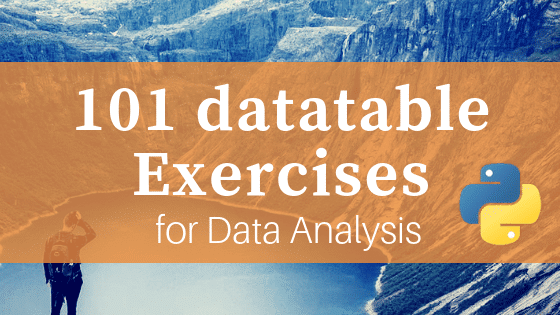## 101 Python datatable Exercises (pydatatable)

Python datatable is the newest package for data manipulation and analysis in Python. It carries the spirit of R’s data.table with similar syntax. It is super fast, much faster than pandas and has the ability to work with out-of-memory data. Looking at the performance it is on path to become a must-use package for data …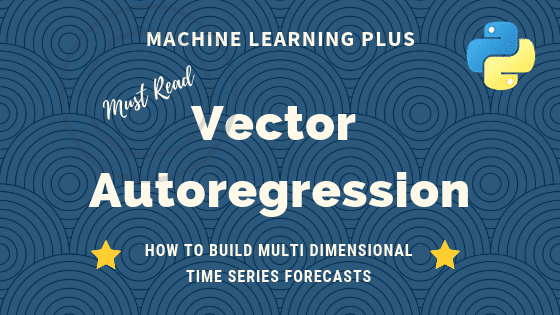## Vector Autoregression (VAR) – Comprehensive Guide with Examples in Python

Vector Autoregression (VAR) is a forecasting algorithm that can be used when two or more time series influence each other. That is, the relationship between the time series involved is bi-directional. In this post, we will see the concepts, intuition behind VAR models and see a comprehensive and correct method to train and forecast VAR …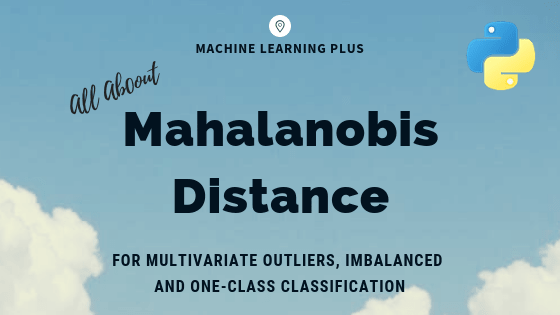## Mahalanobis Distance – Understanding the math with examples (python)

Mahalanobis distance is an effective multivariate distance metric that measures the distance between a point and a distribution. It is an extremely useful metric having, excellent applications in multivariate anomaly detection, classification on highly imbalanced datasets and one-class classification. This post explains the intuition and the math with practical examples on three machine learning use …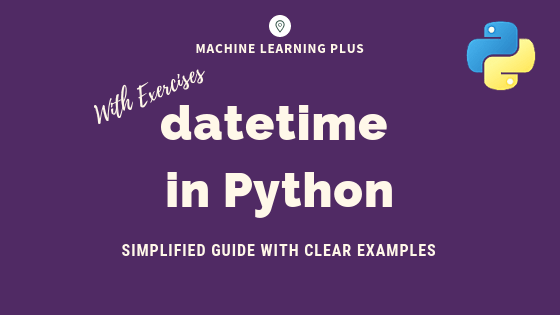## datetime in Python – Simplified Guide with Clear Examples

datetime is the standard module for working with dates in python. It provides 4 main objects for date and time operations: datetime, date, time and timedelta. In this post you will learn how to do all sorts of operations with these objects and solve date-time related practice problems (easy to hard) in Python. datetime in …## Principal Component Analysis (PCA) – Better Explained

Principal Components Analysis (PCA) is an algorithm to transform the columns of a dataset into a new set of features called Principal Components. By doing this, a large chunk of the information across the full dataset is effectively compressed in fewer feature columns. This enables dimensionality reduction and ability to visualize the separation of classes …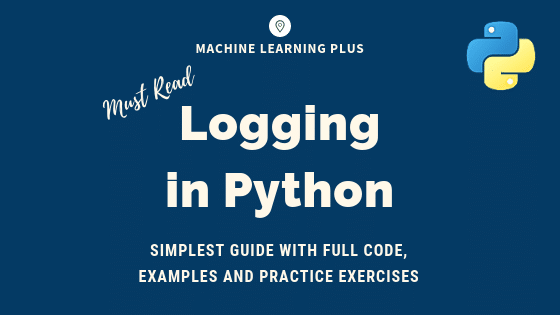## Python Logging – Simplest Guide with Full Code and Examples

The logging module lets you track events when your code runs so that when the code crashes you can check the logs and identify what caused it. Log messages have a built-in hierarchy – starting from debugging, informational, warnings, error and critical messages. You can include traceback information as well. It is designed for small …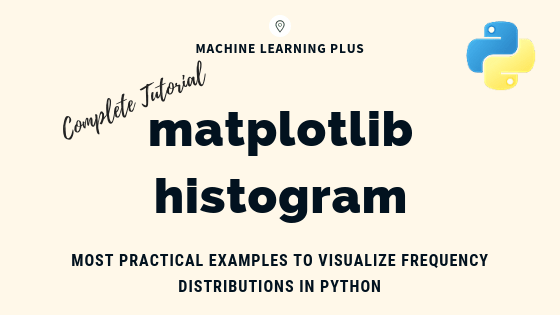## Matplotlib Histogram – How to Visualize Distributions in Python

Matplotlib histogram is used to visualize the frequency distribution of numeric array by splitting it to small equal-sized bins. In this article, we explore practical techniques that are extremely useful in your initial data analysis and plotting. Content What is a histogram? How to plot a basic histogram in python? Histogram grouped by categories in …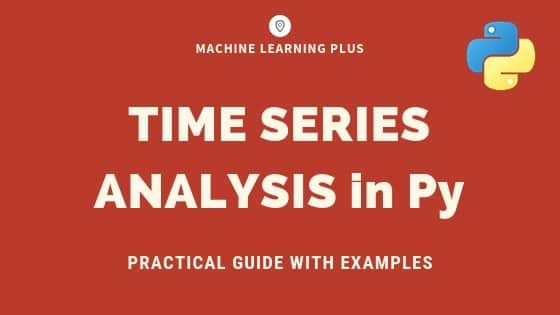## Time Series Analysis in Python – A Comprehensive Guide with Examples

Time series is a sequence of observations recorded at regular time intervals. This guide walks you through the process of analyzing the characteristics of a given time series in python. Time Series Analysis in Python – A Comprehensive Guide. Photo by Daniel Ferrandiz. Contents What is a Time Series? How to import Time Series in …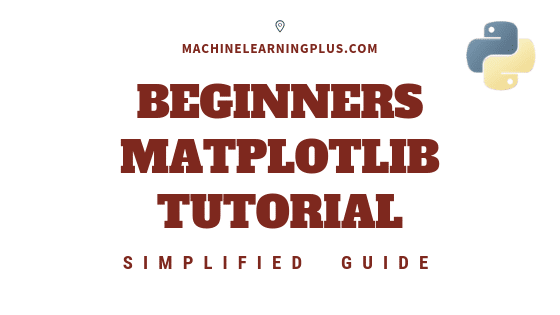## Matplotlib Tutorial – A Complete Guide to Python Plot with Examples

Until next time Until next time This tutorial explains matplotlib’s way of making plots in simplified parts so you gain the knowledge and a clear understanding of how to build and modify full featured matplotlib plots. 1. Introduction Matplotlib is the most popular plotting library in python. Using matplotlib, you can create pretty much any …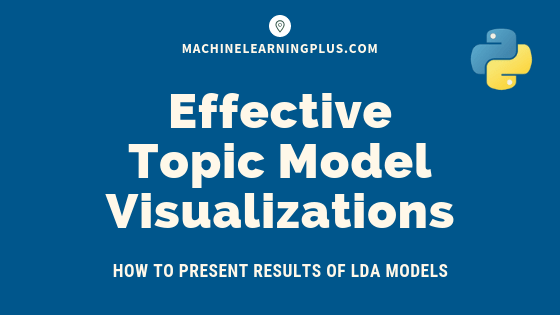## Topic modeling visualization – How to present the results of LDA models?

In this post, we discuss techniques to visualize the output and results from topic model (LDA) based on the gensim package. Topic modeling visualization – How to present the results of LDA models? Contents Introduction Import NewsGroups Dataset Tokenize Sentences and Clean Build the Bigram, Trigram Models and Lemmatize Build the Topic Model Presenting the …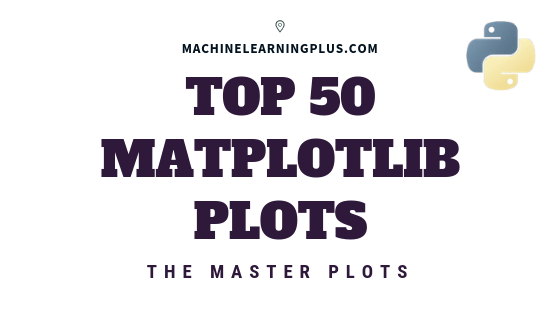## Top 50 matplotlib Visualizations – The Master Plots (with full python code)

A compilation of the Top 50 matplotlib plots most useful in data analysis and visualization. This list lets you choose what visualization to show for what situation using python’s matplotlib and seaborn library. Introduction The charts are grouped based on the 7 different purposes of your visualization objective. For example, if you want to picturize …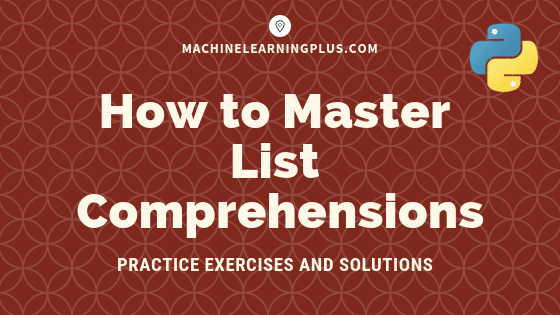## List Comprehensions in Python – My Simplified Guide

List comprehensions is a pythonic way of expressing a ‘For Loop’ that appends to a list in a single line of code. It is an intuitive, easy-to-read and a very convenient way of creating lists. This is a beginner friendly post for those who know how to write for-loops in python but don’t quite understand …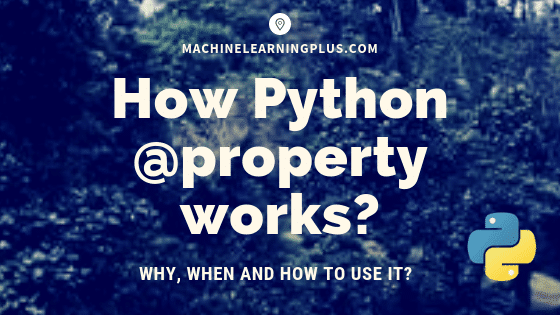## Python @Property Explained – How to Use and When? (Full Examples)

A python @property decorator lets a method to be accessed as an attribute instead of as a method with a ‘()’. Today, you will gain an understanding of when it is really needed, in what situations you can use it and how to actually use it. Contents 1. Introduction2. What does @property do?3. When to …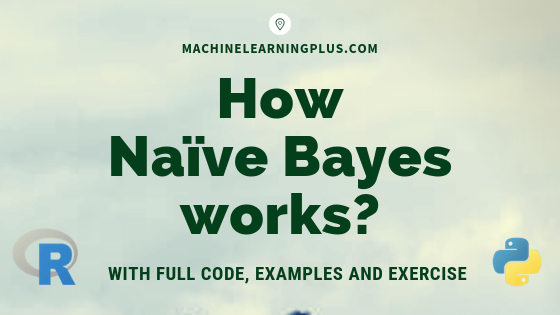## How Naive Bayes Algorithm Works? (with example and full code)

Naive Bayes is a probabilistic machine learning algorithm based on the Bayes Theorem, used in a wide variety of classification tasks. In this post, you will gain a clear and complete understanding of the Naive Bayes algorithm and all necessary concepts so that there is no room for doubts or gap in understanding. Contents 1. …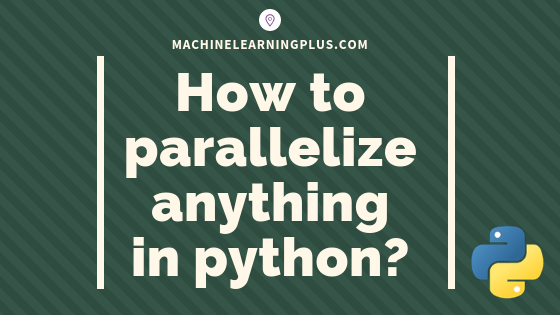## Parallel Processing in Python – A Practical Guide with Examples

Parallel processing is a mode of operation where the task is executed simultaneously in multiple processors in the same computer. It is meant to reduce the overall processing time. In this tutorial, you’ll understand the procedure to parallelize any typical logic using python’s multiprocessing module. 1. Introduction Parallel processing is a mode of operation where …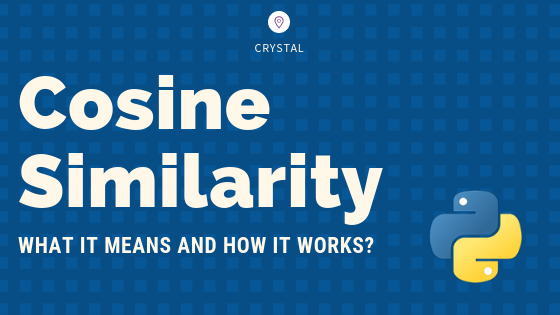## Cosine Similarity – Understanding the math and how it works (with python codes)

Cosine similarity is a metric used to measure how similar the documents are irrespective of their size. Mathematically, it measures the cosine of the angle between two vectors projected in a multi-dimensional space. The cosine similarity is advantageous because even if the two similar documents are far apart by the Euclidean distance (due to the …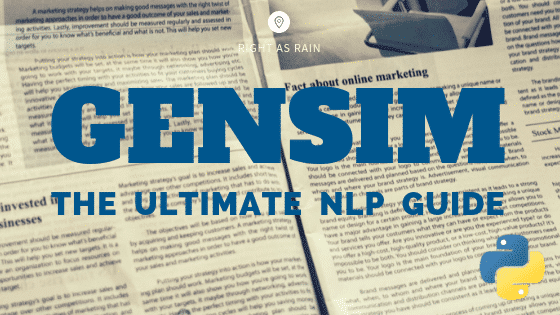## Gensim Tutorial – A Complete Beginners Guide

Gensim is billed as a Natural Language Processing package that does ‘Topic Modeling for Humans’. But it is practically much more than that. It is a leading and a state-of-the-art package for processing texts, working with word vector models (such as Word2Vec, FastText etc) and for building topic models. Gensim Tutorial – A Complete Beginners …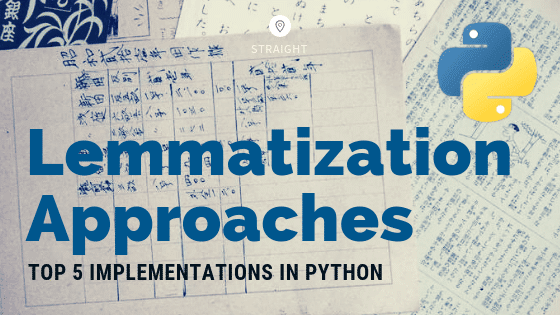## Lemmatization Approaches with Examples in Python

Lemmatization is the process of converting a word to its base form. The difference between stemming and lemmatization is, lemmatization considers the context and converts the word to its meaningful base form, whereas stemming just removes the last few characters, often leading to incorrect meanings and spelling errors. Comparing Lemmatization Approaches in Python. Photo by …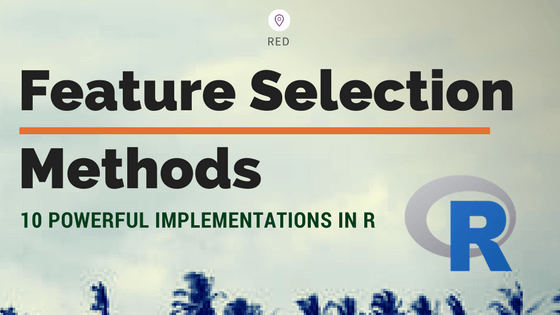## Feature Selection – Ten Effective Techniques with Examples

In machine learning, Feature selection is the process of choosing variables that are useful in predicting the response (Y). It is considered a good practice to identify which features are important when building predictive models. In this post, you will see how to implement 10 powerful feature selection approaches in R. Introduction 1. Boruta 2. …Course Preview

## Machine Learning A-Z™: Hands-On Python & R In Data Science

### Free Sample Videos:#### Machine Learning A-Z™: Hands-On Python & R In Data Science#### Machine Learning A-Z™: Hands-On Python & R In Data Science#### Machine Learning A-Z™: Hands-On Python & R In Data Science#### Machine Learning A-Z™: Hands-On Python & R In Data Science